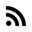# Bit mathematics cookbook

I found this amazing cookbook written by Joel Yliluoma a.k.a bisqwit. Just check it if you are interested in bit operations, and it is highly recommended if you work in embedded engineering. Note Two’s complement is assumed.

Here is an example of Addition without carry

``````// Using subtraction
uint_type a      = original_1;
uint_type b      = original_2;
b = -b;
uint_type result = a - b;

// Using bitwise operations, with XOR
uint_type a      = original_1;
uint_type b      = original_2;
uint_type carry  = a & b;
uint_type result = a ^ b;
while(carry != 0) {
// If you need the mathematical carry from addition,
// check the overflow from this shift.
uint_type shiftedcarry = carry << 1;
carry  = result & shiftedcarry;
result = result ^ shiftedcarry;
}
``````

That guy is sick.